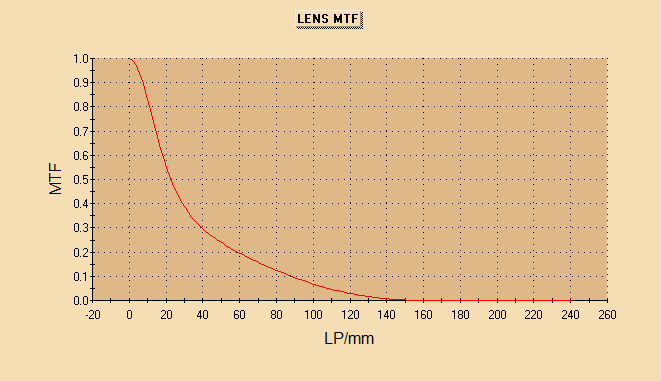# Subroutine for custom 2D Plots

This subroutine can be used to create 2D data plots in FRED:

```Sub Plot2D( ByVal xdata() As Double, _
ByVal ydata() As Double, _
ByVal title As String, _
ByVal xlabel As String, _
ByVal ylabel As String, _
ByVal saveImg As Boolean)

Dim plotNode As Long
Dim plotSeries As Long

plotSeries = AddUser2DPlotSeries( plotNode, xdata(), ydata() )

SetUser2DPlotGraphTitle( plotNode, title )
SetUser2DPlotXaxisTitle( plotNode, xlabel )
SetUser2DPlotYaxisTitle( plotNode, ylabel )

If saveImg Then
SaveUser2DPlotGraphToFile plotNode, GetDocDir() & "\" & title & ".jpg" , 100, False, False
End If

End Sub
```

The following need to be passed to the subroutine:

xdata() as Double

- the array of data points on the x axis

ydata() as Double

- the array of data points on the y axis (must be the same size as xdata)

title as String

- the title to be shown at the top of the 2D plot

xlabel as String

- the label for the x axis

ylabel as String

- the label for the y axis

saveImg as Boolean

- for saving a jpg image of the plot in the same directory as the .frd file.

For example,

```    'Plot the data in 2D Chart using the subroutine defined below
Plot2D(xdata(), ydata(), "LENS MTF", "LP/mm", "MTF", True)
```